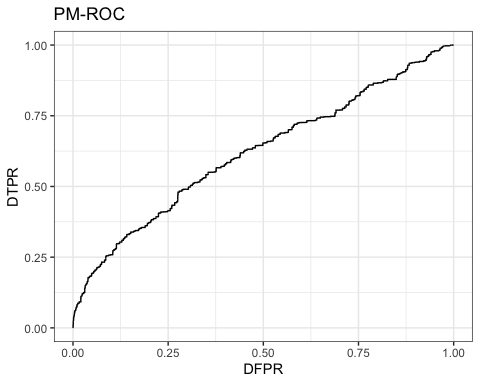# A Guide to R Package DySS

library(DySS)
#> Warning in register(): Can't find generic scale_type in package ggplot2 to
#> register S3 method.

## Univariate DySS

### Preliminaries: How to Prepare Data

Let us consider a typical longitudinal dataset. Suppose that there are $$m$$ subjects, and for the $$i$$th subject, the longitudinal observations are taken at times $$t_{i1},\ldots,t_{in_i}$$ in the design interval $$[T_\text{min},T_\text{max}]$$, where $$n_i$$ is the number of observations for the $$i$$th subject. The corresponding observations at those times are denoted by $$y_{i1},\ldots,y_{in_i}$$. Before applying this package, it is recommended to prepare the dataset in the following format. Data $$y_{ij}$$ will be stored in a matrix as follows. $\mathbf{Y}= \begin{bmatrix} y_{11}&y_{12}&\cdots&\cdots&y_{1n_1}&\text{NA}&\text{NA}\\ y_{21}&y_{22}&\cdots&y_{2n_2}&\text{NA}&\text{NA}&\text{NA}\\ \vdots&\vdots&\cdots&\cdots&\cdots&\cdots&\cdots\\ y_{m1}&y_{m2}&\cdots&\cdots&\cdots&y_{mn_m}&\text{NA}\\ \end{bmatrix}.$

The $$(i,j)$$th element of the matrix is $$y_{ij}$$ if $$j\le n_i$$ and otherwise. Similarly, the observation times will be stored a matrix as follows. $\mathbf{T}= \begin{bmatrix} t_{11}&t_{12}&\cdots&\cdots&t_{1n_1}&\text{NA}&\text{NA}\\ t_{21}&t_{22}&\cdots&t_{2n_2}&\text{NA}&\text{NA}&\text{NA}\\ \vdots&\vdots&\cdots&\cdots&\cdots&\cdots&\cdots\\ t_{m1}&t_{m2}&\cdots&\cdots&\cdots&t_{mn_m}&\text{NA}\\ \end{bmatrix}.$

The number of observations will be stored in a vector, $\mathbf{N}=(n_1,\ldots,n_m)'.$ where the $$i$$ the component is the number of observations for the $$i$$th subject.

The above three are the main components that are needed for the analysis. For some methods that rely on the survival information of the subjects, one will need to provide additional data. The starting times should be stored in a numeric vector $$(E_1,\ldots,E_m)'$$, the survival times should be stored in a numeric vector $$(T_1,\ldots,T_m)'$$, and the survival event indicators should be stored in a logical vector $$(\delta_1,\ldots,\delta_m)'$$. $$S_i$$, $$T_i$$ and $$\delta_i$$ are the starting time, survival time and survival event indicator of the $$i$$th subject respectively.

Besides the observed data, one will also need to specify the following design time parameters. The design time interval $$[T_\text{min},T_\text{max}]$$ specifies the time range of interest in the analysis. The time-varying parameters in the model (e.g., the mean and covariance functions) will be estimated in this range. $$\omega$$ defines the basic time unit which is the smallest time unit in the analysis. all observed times will be rounded to the closest values in the sequence $$T_\text{min},T_\text{min}+\omega,T_\text{min}+2\omega,\ldots,T_\text{max}$$. In the evaluation of control charts, the average time to signal is also expressed in terms of multiples of the basic time unit. Since the values of $$T_\text{min}$$, $$T_\text{max}$$, and $$\omega$$ are user supplied, it may not be always true that $$T_\text{max}-T_\text{min}$$ is a muliple of $$\omega$$. In this case some mild adjustments will be applied to the value of $$\omega$$. Alternatively, one can also specify the total number of basic time unit $$n_\omega$$ in the design interval. In this case the basic time unit $$\omega$$ is given by $$(T_\text{max}-T_\text{min})/(n_\omega-1)$$.

### Step 1: Estimation of Univariate Longitudinal Pattern

We will demonstrate the use of this package using simulated datasets that are provided in this package. First let us load the dataset by the following command.

data("data_example_long_1d")

The IC and OC datasets both consist of 200 subjects.

nrow(data_example_long_1d$data_matrix_IC) #>  200 nrow(data_example_long_1d$data_matrix_OC)
#>  200

The longitudinal observations and observation times are stored in the matrices and , and the number of observations are stored in the vector . For this dataset, we have $$n_i=200$$ for all $$i$$. Then we can apply function to estimate the pattern.

result_pattern<-estimate_pattern_long_1d(
data_matrix=data_example_long_1d$data_matrix_IC, time_matrix=data_example_long_1d$time_matrix_IC,
nobs=data_example_long_1d$nobs_IC, design_interval=data_example_long_1d$design_interval,
n_time_units=data_example_long_1d$n_time_units, estimation_method="meanvar", smoothing_method="local linear", bw_mean=0.1, bw_var=0.1) and are two bandwidth parameters that need to be supplied by the user. Depending on the estimation method, different bandwidth parameters will be required. Here is a list of available methods for estimating the longitudinal pattern and the corresponding required parameters. estimation_method output requireed parameters "meanvar" class: "patn_long_univ_meanvar" hh_mean,hh_var "meanvarcov" class: "patn_long_univ_meanvarcov" hh_mean,hh_var,hh_cov "meanvarcovmean" class: "patn_long_univ_meanvarcov" hh_mean,hh_var,hh_cov "distribution" class: "patn_long_univ_dist" hh_t,hh_y "distributionvarcov" class: "patn_long_univ_distvarcov" hh_t,hh_y,hh_cov In the above example, the function estimates the mean and variance function of the IC data. We can visualize the estimated curves by the following command. plot( c(data_example_long_1d$time_matrix_IC[1:20,]),
c(data_example_long_1d$data_matrix_IC[1:20,]), xlab="Time",ylab="Data", type="p",col="gray",pch=16) lines(result_pattern$grid,result_pattern$mean_est) lines(result_pattern$grid,result_pattern$mean_est+qnorm(0.975)*sqrt(result_pattern$var_est))
lines(result_pattern$grid,result_pattern$mean_est-qnorm(0.975)*sqrt(result_pattern$var_est))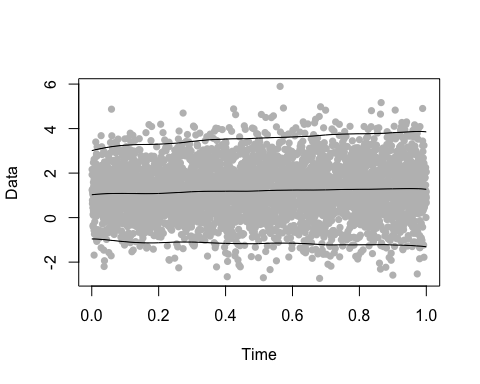### Step 2: Monitor Univariate Longitudinal Data In this step, we apply control charts to monitor if subjects are following the same pattern that we estimated. A signal will be triggered if there is a big deviation between the observed longitudinal data and the estimated pattern. Subjects are monitored by control charts, and the function will calculate the charting statistics for us. Here is an example of how to use this function: chart_IC_output<-monitor_long_1d( data_example_long_1d$data_matrix_IC,
data_example_long_1d$time_matrix_IC, data_example_long_1d$nobs_IC,
pattern=result_pattern,side="upward",chart="CUSUM",
method="standard",parameter=0.2)
chart_OC_output<-monitor_long_1d(
data_example_long_1d$data_matrix_OC, data_example_long_1d$time_matrix_OC,
data_example_long_1d$nobs_OC, pattern=result_pattern,side="upward",chart="CUSUM", method="standard",parameter=0.2) The resulting charting statistics are stored in the matrices , . Here we note that some of the monitoring methods require that other information in addition to the mean and variance is estimated from the data, and therefore can only be applied for some specific type of pattern. Here is a list that gives the required pattern for different monitoring methods. method pattern "standard" "meanvar" or "meanvarcov" "decorrelation" "meanvarcov" "sprint" "meanvarcov" "distribution and standard" "distribution" or "distributionvarcov" "distribution and decorrelation" "distributionvarcov" "distribution and sprint" "distributionvarcov" ### Step 3: Evaluate Performance Next we will demonstrate the use of several functions for evaluating the performance of control charts. The control charts gives a signal if the charting statistics are greater than the threshold called control limit (CL). Given a control limit, we can apply function to calculate the signal times. CL<-4 nind_IC<-nrow(data_example_long_1d$data_matrix_IC)
nind_OC<-nrow(data_example_long_1d$data_matrix_OC) output_signal_times<- calculate_signal_times( chart_matrix=chart_IC_output$chart,
time_matrix=data_example_long_1d$time_matrix_IC, nobs=data_example_long_1d$nobs_IC,
starttime=rep(0,nind_IC),
endtime=rep(1,nind_IC),
design_interval=data_example_long_1d$design_interval, n_time_units=data_example_long_1d$n_time_units,
CL=CL)

The output will tell us whether and when a subject receives a signal.

print(data.frame(
subject=1:10,
signal_time=output_signal_times$signal_times, signal=output_signal_times$signals)[1:10,])
#>    subject signal_time signal
#> 1        1         344   TRUE
#> 2        2          59   TRUE
#> 3        3         315   TRUE
#> 4        4         532   TRUE
#> 5        5         137   TRUE
#> 6        6         409   TRUE
#> 7        7         411   TRUE
#> 8        8         115   TRUE
#> 9        9          60   TRUE
#> 10      10          30   TRUE

In practice, one usually needs to select a control limit such that the average time to signal (ATS) is fixed when the method is applied to IC subjects. We can also apply the function to select control limit , and then calculate the ATS by the function .

CL<-search_CL(
chart_matrix=chart_IC_output$chart, time_matrix=data_example_long_1d$time_matrix_IC,
nobs=data_example_long_1d$nobs_IC, starttime=rep(0,nind_IC), endtime=rep(1,nind_IC), design_interval=data_example_long_1d$design_interval,
n_time_units=data_example_long_1d$n_time_units, ATS_nominal=200, CL_lower=0, CL_upper=10, CL_step=0.5) IC_ATS<- calculate_ATS( chart_matrix=chart_IC_output$chart,
time_matrix=data_example_long_1d$time_matrix_IC, nobs=data_example_long_1d$nobs_IC,
starttime=rep(0,nind_IC),
endtime=rep(1,nind_IC),
design_interval=data_example_long_1d$design_interval, n_time_units=data_example_long_1d$n_time_units,
CL=CL)

OC_ATS<-
calculate_ATS(
chart_matrix=chart_OC_output$chart, time_matrix=data_example_long_1d$time_matrix_OC,
nobs=data_example_long_1d$nobs_OC, starttime=rep(0,nind_OC), endtime=rep(1,nind_OC), design_interval=data_example_long_1d$design_interval,
n_time_units=data_example_long_1d$n_time_units, CL=CL) print(IC_ATS) #>  198.715 print(OC_ATS) #>  103.075 We can evaluate the control charts by ROC curves and PM-ROC curves. We can apply the functions or to perform the evaluation, and the functions and to visualize the curves. The function takes the IC data and OC data separately as different arguments. output_evaluate<-evaluate_control_chart_two_groups( chart_matrix_IC=chart_IC_output$chart,
time_matrix_IC=data_example_long_1d$time_matrix_IC, nobs_IC=data_example_long_1d$nobs_IC,
starttime_IC=rep(0,nind_IC),
endtime_IC=rep(1,nind_IC),
chart_matrix_OC=chart_OC_output$chart, time_matrix_OC=data_example_long_1d$time_matrix_OC,
nobs_OC=data_example_long_1d$nobs_OC, starttime_OC=rep(0,nind_OC), endtime_OC=rep(1,nind_OC), design_interval=data_example_long_1d$design_interval,
n_time_units=data_example_long_1d$n_time_units, no_signal_action="maxtime") The function will draw curves of TPR vs FPR and ATS1 vs ATS0. plot_evaluation(output_evaluate)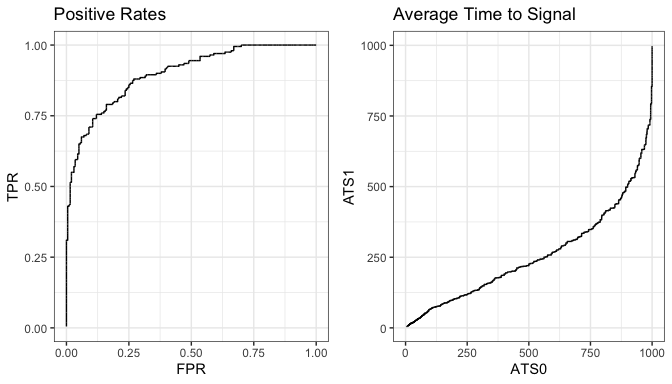The function will draw curves of DTPR vs DFPR which is an evaluation metric proposed in Qiu, Xia and You (2020). plot_PMROC(output_evaluate)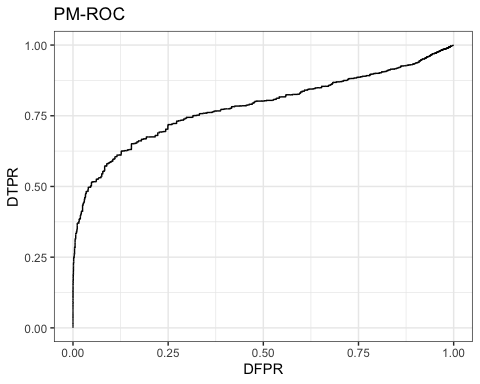## Multivariate DySS ### Preliminaries: How to Prepare Data Similarly, we assume that for the $$i$$th subject, the longitudinal measurements are taken at times $$t_{i1},\ldots,t_{in_i}$$ in the design interval $$[T_\text{min},T_\text{max}]$$, where $$n_i$$ is the number of observations for the $$i$$th subject. The corresponding measurements at those times are denoted by $$\mathbf{y}_{i1},\ldots,\mathbf{y}_{in_i}$$. Each $$\mathbf{y}_{ij}$$ is a $$p$$-dimensional vector $$(y_{ij1},\ldots,y_{ijp})'$$. Observation times and number of observations can be similarly stored in matrix $$\mathbf{T}$$ and $$\mathbf{N}$$. Longitudinal data should be prepared in a 3 dimensional array $$\mathbf{Y}$$, such that the $$(i,j,k)$$th component of $$\mathbf{Y}$$ is $$y_{ijk}$$. ### Step 1: Estimation of Multivariate Longitudinal Pattern We will still demonstrate the use of this package using a simulated dataset that is provided in this package. First let us load the dataset by the following command. data("data_example_long_md") The IC and OC datasets both consist of 200 subjects. nrow(data_example_long_md$data_array_IC)
#>  200
nrow(data_example_long_md$data_array_OC) #>  200 The longitudinal observations and observation times are stored in the 3d arrays and , and the number of observations are stored in the vectors and . For this dataset, we have $$n_i=200$$ for all $$i$$. Then we can apply function to estimate the pattern. We can apply the function to estimate the pattern. result_pattern<-estimate_pattern_long_md( data_array=data_example_long_md$data_array_IC,
time_matrix=data_example_long_md$time_matrix_IC, nobs=data_example_long_md$nobs_IC,
design_interval=data_example_long_md$design_interval, n_time_units=data_example_long_md$n_time_units,
estimation_method="meanvar",
bw_mean=0.10,
bw_var=0.10)

The estimated pattern is then saved in the object . In the analysis, the design interval and all observed times are also discretized into multiples of basic time units, according to the specified arguments and .

Here is a list of available methods for estimating multivariate longitudinal pattern:

Method       Output                             Required arguments
"meanvar"    class: "patn_long_univ_meanvar"    hh_mean, hh_var
"meanvarcov" class: "patn_long_univ_meanvarcov" hh_mean, hh_var, hh_cov

### Step 2: Monitor Multivariate Longitudinal Data

In this step, we apply control charts to monitor if subjects are following the same pattern that we just estimated. A signal will be triggered if there is a big deviation between the observed longitudinal data and the estimated pattern. Subjects are monitored by control charts, and the function will calculate the charting statistics for us. The use is very similar to .

chart_IC_output<-monitor_long_md(
data_array_new=data_example_long_md$data_array_IC, time_matrix_new=data_example_long_md$time_matrix_IC,
nobs_new=data_example_long_md$nobs_IC, pattern=result_pattern, side="upward", method="multivariate EWMA", parameter=0.5,CL=Inf) chart_OC_output<-monitor_long_md( data_array_new=data_example_long_md$data_array_OC,
time_matrix_new=data_example_long_md$time_matrix_OC, nobs_new=data_example_long_md$nobs_OC,
pattern=result_pattern,
side="upward",
method="multivariate EWMA",
parameter=0.5,CL=Inf)

Here is a list of methods that can be applied to monitoring univariate longitudinal data.

Method                Pattern Should Be
"simultaneous CUSUM"  "patn_long_mult_meanvar" or "patn_long_mult_meanvarcov"
"simultaneous EWMA"   "patn_long_mult_meanvar" or "patn_long_mult_meanvarcov"
"multivariate CUSUM"  "patn_long_mult_meanvar" or "patn_long_mult_meanvarcov"
"multivariate EWMA"   "patn_long_mult_meanvar" or "patn_long_mult_meanvarcov"
"decorrelation CUSUM" "patn_long_mult_meanvarcov"
"decorrelation EWMA"  "patn_long_mult_meanvarcov"

### Step 3: Evaluate Performance

Finally, we can evaluate the performance and visualize the results by the same functions , , and .

output_evaluate<-evaluate_control_chart_two_groups(
chart_matrix_IC=chart_IC_output$chart, time_matrix_IC=data_example_long_md$time_matrix_IC,
nobs_IC=data_example_long_md$nobs_IC, starttime_IC=rep(0,nrow(data_example_long_md$time_matrix_IC)),
endtime_IC=rep(1,nrow(data_example_long_md$time_matrix_IC)), chart_matrix_OC=chart_OC_output$chart,
time_matrix_OC=data_example_long_md$time_matrix_OC, nobs_OC=data_example_long_md$nobs_OC,
starttime_OC=rep(0,nrow(data_example_long_md$time_matrix_OC)), endtime_OC=rep(1,nrow(data_example_long_md$time_matrix_OC)),
design_interval=data_example_long_md$design_interval, n_time_units=data_example_long_md$n_time_units,
no_signal_action="maxtime")
plot_evaluation(output_evaluate)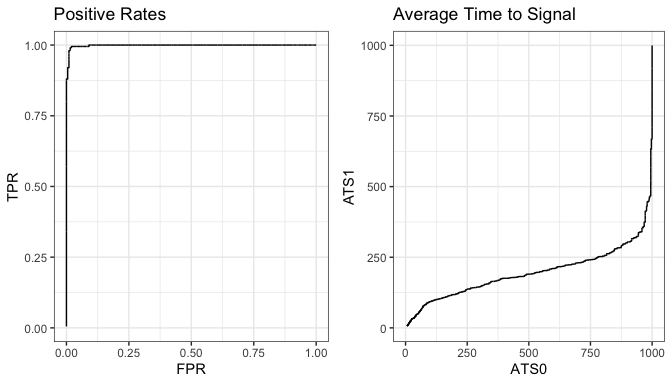plot_PMROC(output_evaluate)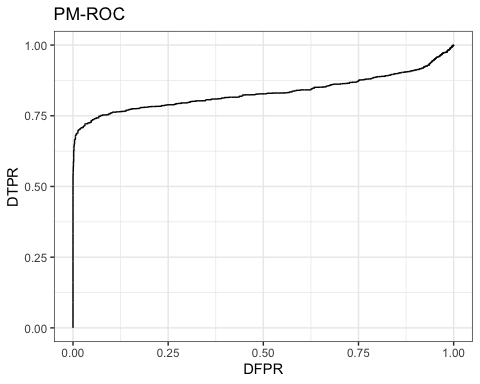## DySS with Survival Outcomes

### Preliminaries: How to Prepare Data

Similarly, we assume that for the $$i$$th subject, the longitudinal measurements are taken at times $$t_{i1},\ldots,t_{in_i}$$ in the design interval $$[T_\text{min},T_\text{max}]$$, where $$n_i$$ is the number of observations for the $$i$$th subject. The corresponding measurements at those times are denoted by $$\mathbf{y}_{i1},\ldots,\mathbf{y}_{in_i}$$. Each $$\mathbf{y}_{ij}$$ is a $$p$$-dimensional vector $$(y_{ij1},\ldots,y_{ijp})'$$. Observation times and number of observations can be similarly stored in matrix $$\mathbf{T}$$ and $$\mathbf{N}$$. Longitudinal data should be prepared in a 3 dimensional array $$\mathbf{Y}$$, such that the $$(i,j,k)$$th component of $$\mathbf{Y}$$ is $$y_{ijk}$$. Additionally, it is also assumed that the dataset contains the survival outcomes of the subjects. A typical survival dataset should include the following three components $$(S_i,T_i,\Delta_i)$$. $$\Delta_i$$ is a binary outcome indicating if an event is observed for the $$i$$th subject. $$S_i$$ is the starting (entry) time of the $$i$$th subject, which usually represents the time when the $$i$$th subject starts to be monitored. $$T_i$$ is the survival time of the $$i$$th subject, which usually represents the time that the $$i$$th subject experiences the event (when $$\Delta_i=1$$) or the time when the $$i$$th subject is lost-to-follow-up (when $$\Delta_i=0$$). The information is organized as three separate vectors, $$=(S_1,S_2,\ldots,S_n)'$$, $$=(T_1,T_2,\ldots,T_n)'$$, and $$=(\Delta_1,\Delta_2,\ldots,\Delta_n)'$$.

### Step 1: Estimation of the Risk Pattern

We will still demonstrate the use of this package using a simulated dataset that is provided in this package. First let us load the dataset by the following command.

data("data_example_long_surv")

The longitudinal observations are stored in the 3d array . The observation times for the longitudinal data are stored in the matrix . The number of observations are stored in the vector . The starting times, survival times and survival events are stored in the vectors , , and . We can apply the function to estimate the pattern.

result_pattern<-estimate_pattern_long_surv(
data_array=data_example_long_surv$data_array_IC, time_matrix=data_example_long_surv$time_matrix_IC,
nobs=data_example_long_surv$nobs_IC, starttime=data_example_long_surv$starttime_IC,
survtime=data_example_long_surv$survtime_IC, survevent=data_example_long_surv$survevent_IC,
design_interval=data_example_long_surv$design_interval, n_time_units=data_example_long_surv$n_time_units,
estimation_method="risk",
smoothing_method="local linear",
bw_beta=0.05,
bw_mean=0.1,
bw_var=0.1)

The estimated pattern is then saved in the object . Currently, only the method is available in the package, and the smoothing method should be one of or .

### Step 2: Monitor Multivariate Longitudinal Data for Risks of Survival Outcomes

In this step, we apply control charts to monitor if subjects are at high risks of the survival outcomes of interest. A signal will be triggered if the estimated risk is higher compared to the risk pattern estimated from IC data. Subjects are monitored by control charts, and the function will calculate the charting statistics for us. The use is very similar to .

result_monitoring<-monitor_long_surv(
data_array_new=data_example_long_surv$data_array_IC, time_matrix_new=data_example_long_surv$time_matrix_IC,
nobs_new=data_example_long_surv$nobs_IC, pattern=result_pattern, method="risk", parameter=0.5) ### Step 3: Evaluate Performance Finally, we can evaluate the performance and visualize the results by the same functions , , and . output_evaluate<-evaluate_control_chart_one_group( chart_matrix=result_monitoring$chart,
time_matrix=data_example_long_surv$time_matrix_IC, nobs=data_example_long_surv$nobs_IC,
starttime=rep(0,nrow(data_example_long_surv$time_matrix_IC)), endtime=rep(1,nrow(data_example_long_surv$time_matrix_IC)),
status=data_example_long_surv$survevent_IC, design_interval=data_example_long_surv$design_interval,
n_time_units=data_example_long_surv\$n_time_units,
no_signal_action="maxtime")
plot_evaluation(output_evaluate)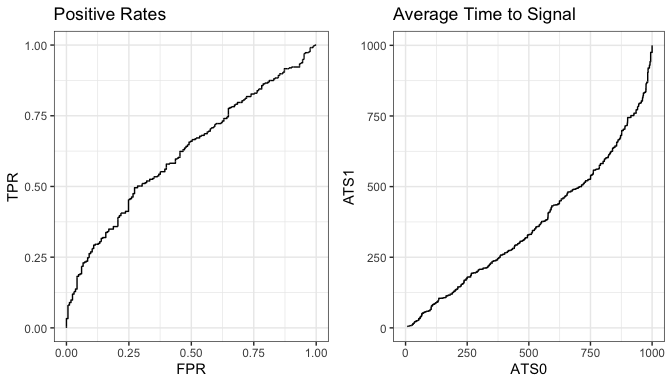plot_PMROC(output_evaluate)